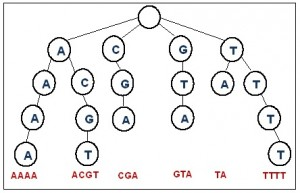# Trie Data Structure

In Computer Science, a trie is a data structure that is also known as a digital search tree or a prefix tree. It can be used for fast retrieval on large data sets such as looking up words in a dictionary. The term trie was invented from the phrase ‘Information Retrieval’ by Fredkin(1960).

As a prefix tree, the trie is an ordered tree used to represent a set of words (or strings) over a finite alphabet set. It allows words with common prefixes to use the same prefix data and stores only the end of the words to indicate different words. Each node in the trie is labeled with one character except the root node which is an empty node. The maximum number of children that a particular node in the trie can have is equal to the number of characters in the alphabet set used for the trie. The children of a node are alphabetically ordered and sibling nodes must represent distinct characters. The node which contains the last character of a word is called a leaf node.  The path from the root to a leaf node represents a word in the trie.

Consider the set C = { ‘A’, ’C’, ’G’, ’T’}, then a trie for this set can potentially have 4 children for each node; one corresponding to each letter. The following figure shows a trie for 6 different strings composed from C. The strings are: ‘AAAA’, ‘ACGT’, ‘CGA’, ‘GTA’, TA’, and ‘TTTT’. Note that ‘A’, ‘AA’, ‘AAA’, ‘AC’, ‘CG’, ‘ACG’, ‘C’, ‘G’, ‘GT’, ‘T’, ‘TT’, ‘TTT’ are all common prefixes. Also, the height of the trie is the same as the longest word in the trie which is 4 in this case.A standard trie data structure can be developed from a single node class with 3 attributes:

1. A character ch to store the letter representing the node
2. An array of nodes children to represent the children of the node, and
3. A Boolean leafNode to mark the end of the word.
```Class Node{
Char ch;
Node [] children;
Boolean leafNode;
}```

To implement a trie for the previous example, we start with the root node of the trie. Therefore, we instantiate a node object root  by using the following Node constructor:

```Node (){
ch = ‘’ ;  //empty character
children = new Node ;  //null nodes
leafNode = false;
}```

We also need to determine the position for each character in the array so that they are alphabetically ordered. I.e.

`children=’A’; children=’C’; children=’G’; children=’T’;`

Two main functions are needed with a trie data structure: Search and Insert. The following pseudo codes provide the algorithms for both functions.

Void INSERT (String word)

1. Make current node points to the root of the trie.
2. For each character c in word:
1. Get the pre-determined position for c in the children array
2. If the children node of the current node has nothing at that position, insert c
3. Make current node points to its child node whose index is the same as position.
3. Set leafNode to true to indicate insertion of word into the trie.

Boolean SEARCH (String word)

1. Make current node points to the root of the trie.
2. For each character c in word:
1. If there is nothing in current node, return false.
2. Get the pre-determined position for c in the children array
3. Make current node points to its child node whose index is the same as position.
3. If current node is not empty and leafNode is false, return false.
4. Return true to indicate that word was found in the trie.

The trie data structure is often overlooked by developers because of the time it takes to build. For a text of length n, inserting words into the trie requires (n2) time overall. Space needed for a trie is also quadratic which can be another drawback. On the other hand, searching a trie is fast. In the worst case it is  O(m)where m is the length of the word to search for.

Categories: Uncategorized